# 2 Two firms Intel and AND in the CPU market have combined demand given by QE...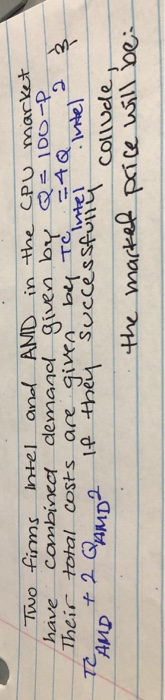2 Two firms Intel and AND in the CPU market have combined demand given by QE 100-P Their total costs are given by To =4Q Intel Intel If they successfully collude the market price will be TE AMD + 2 QAMD²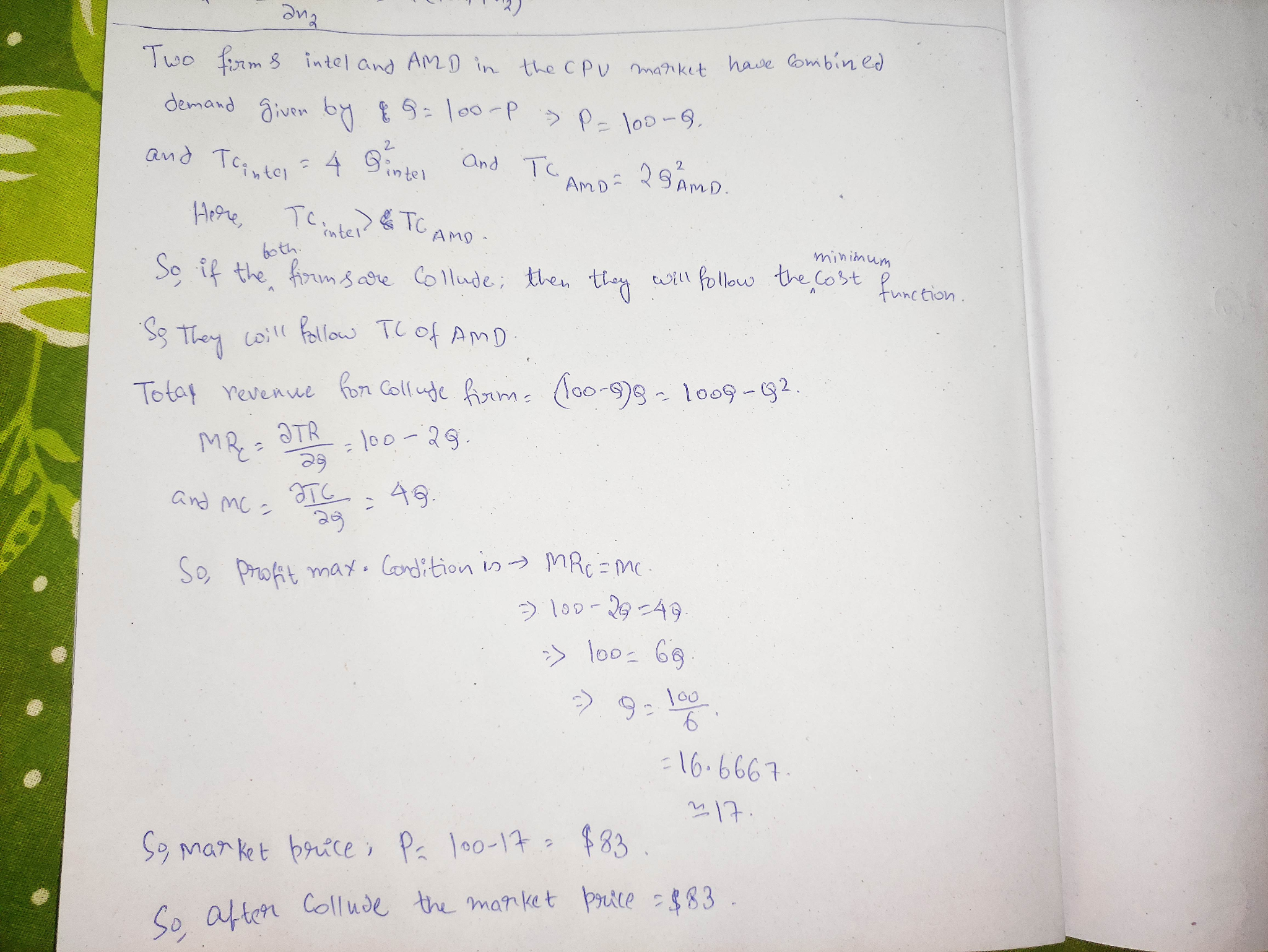##### Add Answer of: 2 Two firms Intel and AND in the CPU market have combined demand given by QE...
Similar Homework Help Questions
• ### Two firms Intel and AMD in the CPU market have combined demand given by Q =...

Two firms Intel and AMD in the CPU market have combined demand given by Q = 100 - P. Their total costs are given by TC Intel = 4 Q Intel + 2 Q Intel^2 and TC AMD = 4 Q AMD + 2 Q AMD^2. If they successfully collude, the market price will be??

• ### Two firms Intel and AMD in the CPU market have combined demand given by Q =...

Two firms Intel and AMD in the CPU market have combined demand given by Q = 100 - 2 P. Their total costs are given by TC Intel = 2 Q Intel + Q^2 Intel and TC AMD = 2 Q AMD + Q^2AMD. If they cannot successfully collude and instead produce where the market price equals marginal cost, the market price will be: Answers: 66 66.67 34 32 98

• ### Te Intel Intel and + Q2 Two firms intel and AMD in the CPU market have...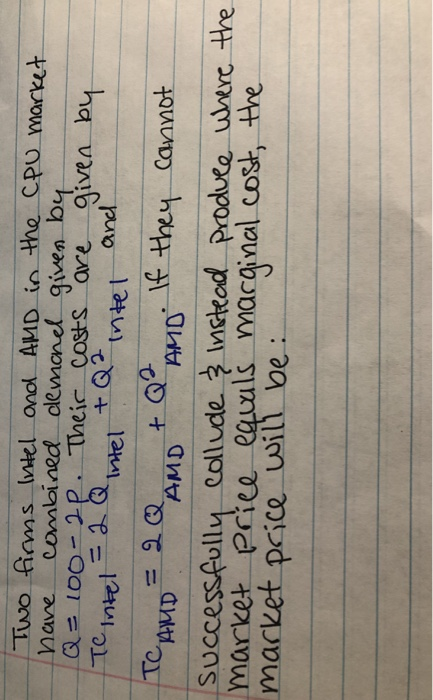Te Intel Intel and + Q2 Two firms intel and AMD in the CPU market have combined demand given by Q = 100-2p. Their costs are given by = 2 Q + Q² Intel TC AMD = 2Q If they cannot AMD AMD successfully collude & Instead produce where the market Price equals marginal cost the market price will be

• ### Suppose we have a market demand Q = 18 – P and a cost C(Q) 9)...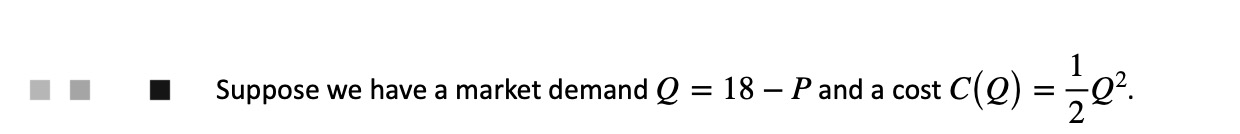Suppose we have a market demand Q = 18 – P and a cost C(Q) 9) = 3Q?. Suppose a second firm enters the market described in question 1 (market demand is 1 still Q = 18 – P) with the same cost (cle) = 109. a. If the two firms successful collude what is the equilibrium market quantity and price? b. If the two firms successfully collude what is the joint profit? C. What do we call a collusion...

• ### Suppose that the firms in the Cournot oligopoly decide to collude. The corresponding demand curve for...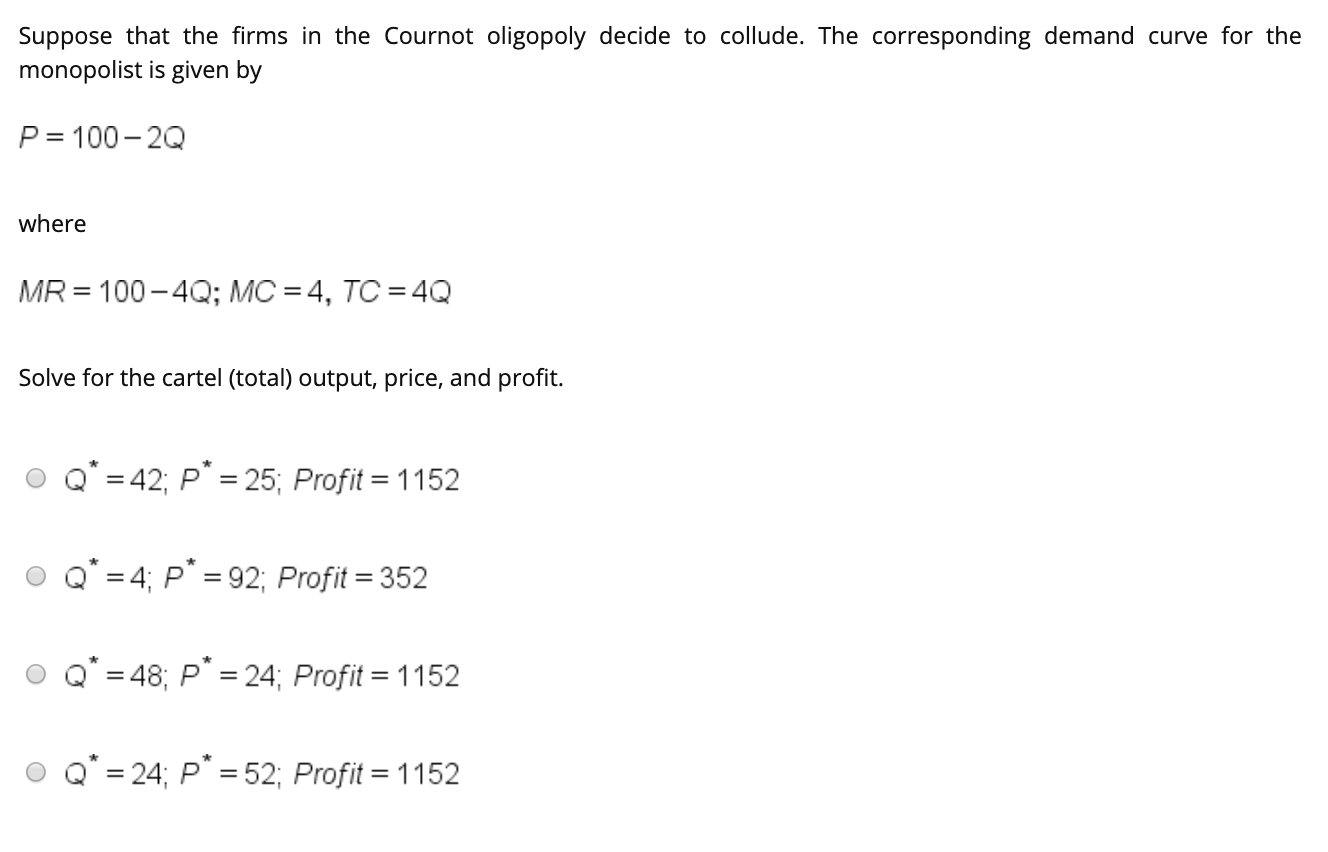Suppose that the firms in the Cournot oligopoly decide to collude. The corresponding demand curve for the monopolist is given by P= 100-20 where MR = 100-4Q; MC = 4, TC = 4Q Solve for the cartel (total) output, price, and profit. O Q* = 42; p* = 25; Profit = 1152 O Q* = 4; p* = 92; Profit = 352 O Q* = 48; P* = 24; Profit = 1152 O Q* = 24; p* = 52; Profit...

• ### Suppose a second firm enters the market described in question 1 (market demand is still Q...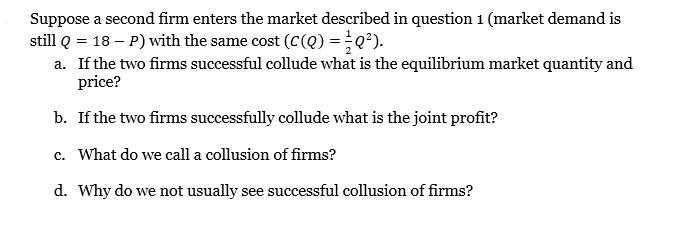Suppose a second firm enters the market described in question 1 (market demand is still Q = 18 – P) with the same cost (CQ) = Q?). a. If the two firms successful collude what is the equilibrium market quantity and price? b. If the two firms successfully collude what is the joint profit? c. What do we call a collusion of firms? d. Why do we not usually see successful collusion of firms?

• ### In a perfect competitive market, industry demand is P = 850 – 2Q, and industry supply is P = 250 + 4Q (supply is the sum of the marginal cost curves of the firms in the industry)

In a perfect competitive market, industry demand is P = 850 – 2Q, and industry supply is P = 250 + 4Q (supply is the sum of the marginal cost curves of the firms in the industry). Assume that all the firms collude to form a single monopoly firm. There is no change in the demand or cost conditions of the industry. What are the economic effects (price and quantity) of such a change in industry structure?

• ### Two firms compete in a market with demand given by D(p) = 100 − p, where...

Two firms compete in a market with demand given by D(p) = 100 − p, where p is denoted in cents (p=100 is 1 dollar). Firms can only charge prices in whole cents – i.e. p can only take integer values, and not values like 1.5. Marginal costs for each firm are given by MC=10. Firms compete by simultaneously choosing prices. When prices are equal, each firm gets one half of total demand. b. Find all the Nash equilibria of...

• ### Suppose two firms cannot collude and compete in the Cournot Model. Market demand is Q =...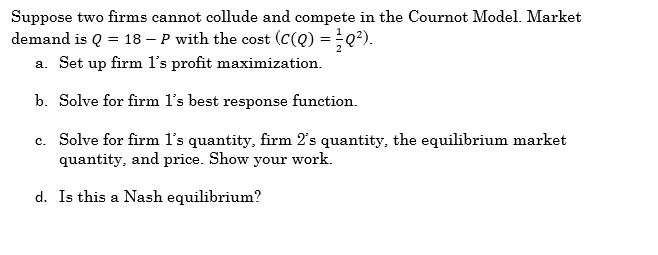Suppose two firms cannot collude and compete in the Cournot Model. Market demand is Q = 18 – P with the cost (c(Q) =*Q). a. Set up firm l's profit maximization. b. Solve for firm l's best response function. c. Solve for firm l's quantity, firm 2's quantity, the equilibrium market quantity, and price. Show your work. d. Is this a Nash equilibrium?

• ### uestion 7 O out of 2 points Two identical firms compete as a Coumot dopoly. The...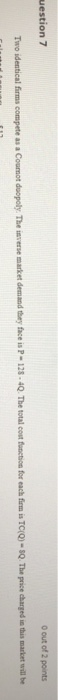uestion 7 O out of 2 points Two identical firms compete as a Coumot dopoly. The inverse market demand they face is P-128 - 4Q. The total cost function for each firm is TCQ) - Q. The price charged in this market will be

Free Homework App